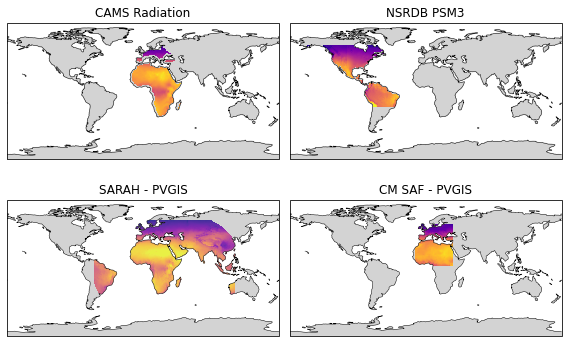Satellite-derived irradiance data has several benefits over ground-measured irradiance despite its lower accuracy. The main advantages include a large geographical coverage (continents), long-timespan (multiple decades), and low cost.

For these reasons, almost all solar projects utilize satellite-derived irradiance data at some stage of the development, whether it be for pre-feasibility site assessment or for calculating the profitability of a project. Fortunately, there are numerous free satellite-derived irradiance datasets available.

Now you may rightfully wonder how these different datasets differ and if there is any difference in their outputs. So in this blog post, we’ll plot the long-term average irradiance for four different irradiance providers.

## Map data#

The data used to generate the maps can be freely downloaded from the links in the table below. Note that the data is based on different time frames, ranging from 10 to 23 years.

Source of map data

Timespan

Unit

SARAH (PVGIS)

2005-2016

W/m\$^2\$/year

CM SAF (PVGIS)

2007-2016

W/m\$^2\$/year

NSRDB PSM3 (NREL)

1998-2016

kWh/m\$^2\$/day

CAMS (SoDa)

2005-2017

kWh/m\$^2\$/year

```# Import necessary packages
import matplotlib.pyplot as plt
import matplotlib.patches as mpatches
import pandas as pd
import numpy as np
import cartopy
# In order for rasterio to include the necessary GDAL library
# install rasterio with conda: conda install -c conda-forge rasterio
import rasterio
from rasterio.plot import show
import cartopy.crs as ccrs
```

Import the raster map data using the python library rasterio.

```sarah = rasterio.open('Data/sarah_ghi_annual.asc')
cmsaf = rasterio.open('Data/cm_saf_ghi_annual.asc')
nsrdb = rasterio.open('Data/nsrdb_psm3_ghi_annual.tif')
cams_eu = rasterio.open('Data/cams_ghi_annual_europe.tif')
cams_af = rasterio.open('Data/cams_ghi_annual_africa.tif')
# Necessary to mask zeros, as 'nodata' attribute is set incorrectly in the tif file
```

## Plot maps#

The map is generated using cartopy and matplotlib. Cartopy makes it seamless to plot countries, borders, and other geographical features.

```kwh_to_w = 1000/8760
vmin, vmax = 800, 2500

crs = cartopy.crs.PlateCarree()

fig, axes = plt.subplots(
nrows=2, ncols=2, figsize=(8, 8),
sharex=True, sharey=True, subplot_kw={'projection': crs})

cams_eu_extent = [cams_eu.bounds, cams_eu.bounds, cams_eu.bounds, cams_eu.bounds]
cams_af_extent = [cams_af.bounds, cams_af.bounds, cams_af.bounds, cams_af.bounds]
rasterio.plot.show(cams_eu_masked, extent=cams_eu_extent, ax=axes[0,0], cmap='plasma', zorder=2, vmin=vmin, vmax=vmax)
rasterio.plot.show(cams_af_masked, extent=cams_af_extent, ax=axes[0,0], cmap='plasma', zorder=2, vmin=vmin, vmax=vmax)

axes[0,1].set_title('NSRDB PSM3')
nsrdb_extent = [nsrdb.bounds, nsrdb.bounds, nsrdb.bounds, nsrdb.bounds]
rasterio.plot.show(nsrdb, extent=nsrdb_extent, ax=axes[0,1], cmap='plasma', zorder=2, vmin=vmin/365, vmax=vmax/365)

axes[1,0].set_title('SARAH - PVGIS')
sarah_extent = [sarah.bounds, sarah.bounds, sarah.bounds, sarah.bounds]
rasterio.plot.show(sarah, extent=sarah_extent, ax=axes[1,0], cmap='plasma', zorder=2, alpha=0.8, vmin=vmin*kwh_to_w, vmax=vmax*kwh_to_w)

axes[1,1].set_title('CM SAF - PVGIS')
cmsaf_extent = [cmsaf.bounds, cmsaf.bounds, cmsaf.bounds, cmsaf.bounds]
ax = rasterio.plot.show(cmsaf, extent=cmsaf_extent, ax=axes[1,1], cmap='plasma', zorder=2, vmin=vmin*kwh_to_w, vmax=vmax*kwh_to_w)

for ii, ax in enumerate(axes.flatten()):
ax.coastlines(edgecolor='black', lw=0.5, zorder=3)
ax.set_extent([-180, 180, -90, 90])
if ii > 1:  # Only plot OCEAN layer for PVGIS